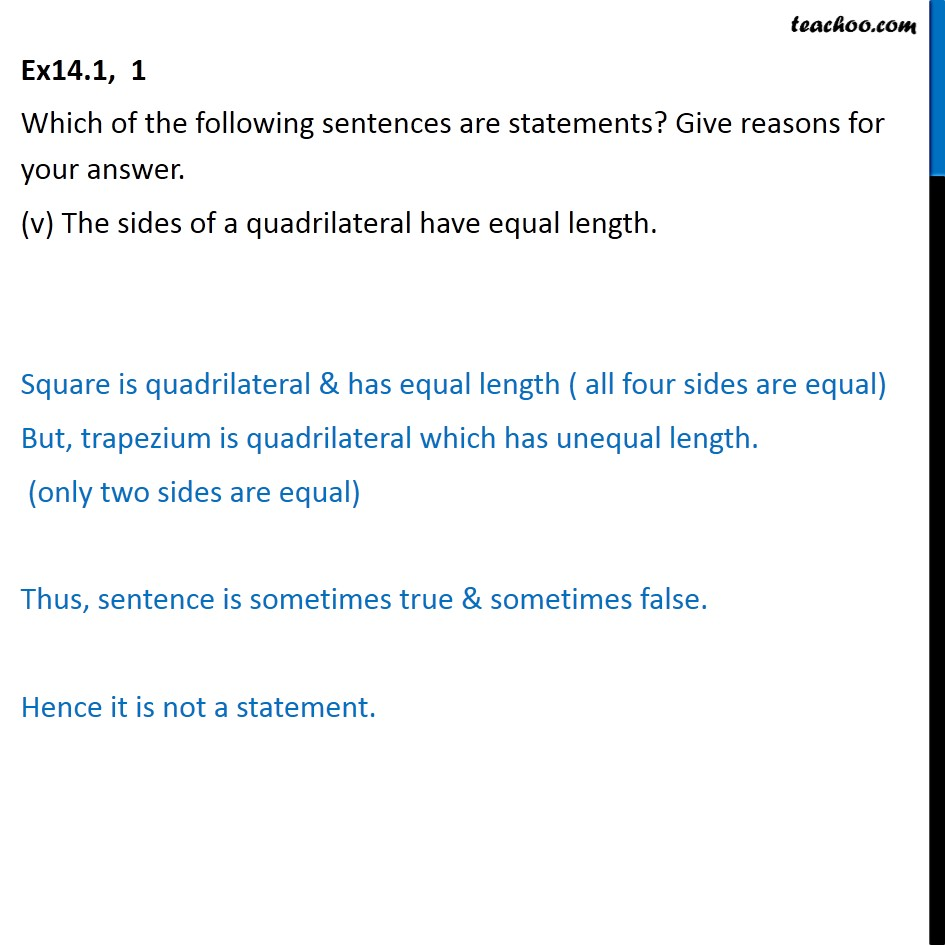Statements

Mathematical Reasoning
Serial order wiseLearn in your speed, with individual attention - Teachoo Maths 1-on-1 Class

### Transcript

Ex14.1, 1 Which of the following sentences are statements? Give reasons for your answer. (v) The sides of a quadrilateral have equal length. Square is quadrilateral & has equal length ( all four sides are equal) But, trapezium is quadrilateral which has unequal length. (only two sides are equal) Thus, sentence is sometimes true & sometimes false. Hence it is not a statement.